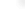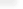## Delay Differential Equations

Mathematica 7 expands Mathematica's broad numerical differential equation capabilities by adding delay differential equations (DDE). Using powerful new automated algorithms, Mathematica 7 for the first time makes it possible to solve DDEs directly from their natural mathematical specification, without the need for manual preprocessing.

Related Functions